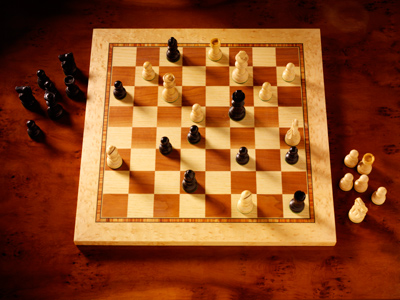A chessboard is 8 squares wide and 8 squares long so it has 64 squares altogether.

# 8 Times Table

In KS1 Maths and Numeracy children will be exposed to the times tables as early as possible in Year 1. The 1, 2, 5 and 10 times tables are taught first before the 3, 4, 6, 7, 8 and 9 times tables follow. By the end of Year 2 children are often fluent in their times tables up to 10.

This quiz will test children's familiarity with their eight times table, which will help them in studying maths in Years 1 and 2.

Do you know your eight times table? This one can be tricky so good luck!

1.
8 x 1 =
0
16
8
10
2.
4 x 8 =
30
24
36
32
3.
8 x 10 =
80
88
72
76
Remember, to times a number by 10 just add a 0 to the end of it
4.
6 x 8 =
44
48
56
32
5.
2 x 8 =
24
16
14
18
If you double 8 you get 16
6.
8 x 8 =
60
72
64
56
There are 64 squares on a chessboard
7.
5 x 8 =
40
48
32
44
8.
3 x 8 =
24
16
32
30
3 x 8 is the same as 8 + 8 + 8
9.
8 x 0 =
8
0
1
10
Any number times 0 = 0
10.
8 x 7 =
60
64
48
56